# Wold decomposition

A decomposition introduced by H. Wold in 1938 (see [a7]); see also [a5], [a8]. Standard references include [a6], [a3].

The Wold decomposition of a (weakly) stationary stochastic process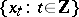,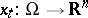, provides interesting insights in the structure of such processes and, in particular, is an important tool for forecasting (from an infinite past).

The main result can be summarized as:

1) Every (weakly) stationary processcan uniquely be decomposed as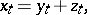where the stationary processes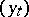andare obtained by causal linear transformations of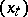(where "causal" means that, e.g.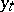, only depends on,),and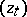are mutually uncorrelated,is linearly regular (i.e. the best linear least squares predictors converge to zero, if the forecasting horizon tends to infinity) andis linearly singular (i.e. the prediction errors for the best linear least squares predictors are zero).

2) Every linearly regular processcan be represented as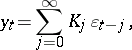(a1)whereis white noise (i.e.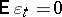,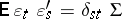) andis obtained by a causal linear transformation of.

The construction behind the Wold decomposition in the Hilbert spacespanned by the one-dimensional process variablesis as follows: If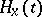denotes the subspace spanned by, then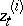is obtained from projectingon the space, andis obtained as the perpendicular by projectingon the space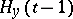spanned by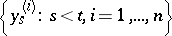. Thusis the innovation and the one-step-ahead prediction error foras well as for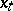.

The implications of the above-mentioned results for (linear least squares) prediction are straightforward: Sinceandare orthogonal and sinceis the direct sum of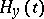and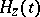, the prediction problem can be solved for the linearly regular and the linearly singular part separately, and for a linearly regular process,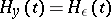implies that the best linear least squares-step ahead predictor foris given by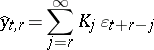and thus the prediction error is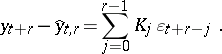Thus, when the representation (a1) is available, the prediction problem for a linearly regular process can be solved.

The next problem is to obtain (a1) from the second moments of(cf. also Moment). The problem of determining the coefficients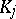of the Wold representation (a1) (or, equivalently, of determining the corresponding transfer function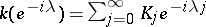) from the spectral density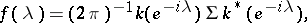(a2)

(where thedenotes the conjugate transpose) of a linearly regular process, is called the spectral factorization problem. The following result holds:

3) A stationary processwith a spectral density, which is non-singular-a.e., is linearly regular if and only if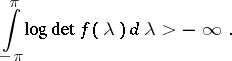In this case the factorization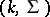in (a2) corresponding to the Wold representation (a1) satisfies the relation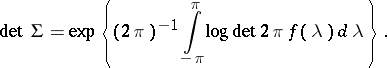The most important special case is that of rational spectral densities; for such one has (see e.g. [a4]):

4) Any rational and-a.e. non-singular spectral densitycan be uniquely factorized, such that(the extension of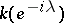to) is rational, analytic within a circle containing the closed unit disc,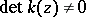,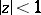,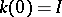(and thus corresponds to the Wold representation (a1)), and. Then (a1) is the solution of a stable and miniphase ARMA or a (linear) finite-dimensional state space system.

Evidently, the Wold representation (a1) relates stationary processes to linear systems with white noise inputs. Actually, Wold introduced (a1) as a joint representation for AR and MA systems (cf. also Mixed autoregressive moving-average process).

The Wold representation is used, e.g., for the construction of the state space of a linearly regular process and the construction of state space representations, see [a1], [a4]. As mentioned already, the case of rational transfer functions corresponding to stable and miniphase ARMA or (finite-dimensional) state space systems is by far the most important one. In this case there is a wide class of identification procedures available, which also give estimates of the coefficientsfrom finite data(see e.g. [a4]).

Another case is that of stationary long memory processes (see e.g. [a2]). In this case, in (a1),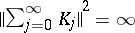, so thatis infinity at frequency zero, which causes the long memory effect. Models of this kind, in particular so-called ARFIMA models, have attracted considerable attention in modern econometrics.